# 8. Asset Schedule (Part 2)

## 8.1 – Hello depreciation

We closed the previous chapter with our first balance sheet projection, i.e. the gross block. This chapter will complete the asset schedule and plug the numbers back to the P&L and Balance sheet.

At this stage, here is how the asset schedule looks  –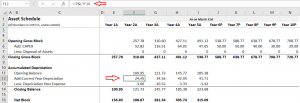The gross block looks tidy. We will now have to work our way through depreciation. The biggest challenge with forecasting the accumulated depreciation is getting the current year depreciation number, which, as you realise, flows from the P&L statement.

Here is the snapshot of current year depreciation as stated in the P&L –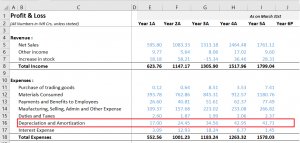Investors are often confused about these two depreciation numbers, i.e. the accumulated depreciation stated in the balance sheet and the current year depreciation number displayed in the P&L (on the expense side).

The current year depreciation stated in the P&L is the depreciation value (in Rupee terms) applicable only for the financial year under consideration. The company’s finance team calculates the current year depreciation by factoring in all the assets (gross block) on its books. The current year depreciation stated in the P&L changes for each year based on how the gross block changes.

On the other hand, the accumulated depreciation in the balance sheet is on a cumulative basis. The depreciation number gets rolled over on year on year basis. In other words, the current year depreciation (from P&L) gets added over to the next year depreciation, thus forming the accumulated depreciation for this year.

Don’t worry if you find this confusing; you will understand this better shortly, but for now, I want to you think about the direction we are heading in.

We have already projected the Gross block number. If we can project the current year depreciation number in the P&L, we can apply the base rule again in the asset schedule and forecast the accumulated depreciation number.

After we forecast the accumulated depreciation, we can also calculate the netblock of the company. Finally, the net block number from the asset schedule flows back to the balance sheet.

By carrying out the above steps, we achieve two things –

• Project the current year depreciation number in P&L
• Get the projected netblock number in the asset schedule, which gets plugged back to the balance sheet

Everything depends on techniques to forecast the current year depreciation.

Before we proceed, a slight but relevant digression 😊

I want you to think about the following situation. Assume you are a freelance photographer with a variable monthly income. Your income depends on the work that comes your way.  You are also responsible for managing your household expenses.Your monthly income for October is 25K, of which you spend 3K on entertainment.

The next month you earn 30K. How much do you think you should spend on entertainment for the month of November?

The easiest way to do this is to spend in the same proportion as you spent in October.

In October –

3000/25000

= 12%

Hence for September,

12% * 30000

= 3600

We have used the method of proportions here. I want to extend this thought and project depreciation for the current year.

For Year 5, Depreciation and Amortization stated in P&L is 41.71Cr. The gross block, as stated in the balance sheet for Year 5, is 538.76Cr. The projected Gross Block for Year 6 is 588.77 Crs. Given this, what do you think will be depreciation for Year 6?

Let us apply the proportion technique.

For gross block worth 538.76Cr, the company reported depreciation of 41.71Cr, which means –

41.71/538.76

= 7.74%

For Year 6, the gross block value is 588.77 Crs, so what is the depreciation given the same proportion?

7.74% * 588.77

= 45.58Cr

With this, we can estimate that the depreciation for the next year would be 45.58Crs. Remember, this number flows into the P&L.

The depreciation value will remain the same at 7.74%.

You can extend this a bit more. Instead of taking the previous year’s proportion and assuming the same proportion will hold for the next year, you can calculate the depreciation to gross block ratio for all the historical years and then take the five years rolling average for the future years.

It will look something like this –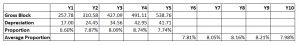Y6 = Average of Y1 to Y5

Y7 = Average of Y2 to Y6

Y8 = Average of Y3 to Y7

So on and so forth.

You can choose either technique; I’ll stick to the first technique for simplicity.

Of course, the 3rd alternative is to dig deep into gross block and get into the accountant shoe to figure the exact depreciation value; you can do that if you have a strong accounting background.

Going back to the P&L with the 1st technique, I can directly input the formula –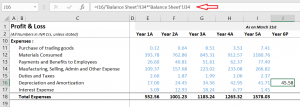As you can see, in cell J16, which points to the Depreciation expense for Year 6, I’ve divided the depreciation amount stated in P&L for year five over the gross block stated in the balance sheet for year 5. The result of this division is the depreciation proportion, which I then multiply by the gross block projected for Year 6.

The resulting value is the depreciation amount for Year 6. Note, this is a projection that we are making. I can extend the same math to all the future years and get the depreciation expense for the year.

By the way, we also made our first P&L projection, so it’s a tiny baby step in our financial modelling journey 😊

At this point, my P&L looks like this –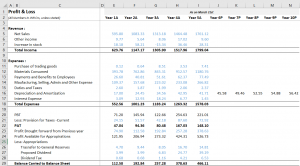Alright, with this in place, let’s go back to the asset schedule.

## 8.2 – Accumulated depreciation

Some of you may have guessed the next few steps already.  It is pretty simple, we go back to the asset schedule sheet and plug in the current year depreciation number from P&l. Once we have that, we use the base rule to complete the accumulated depreciation.

Here is how I’ve plugged the number from P&L –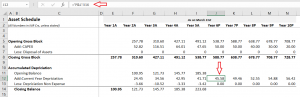We have the closing balance for Y5, i.e. 223.68Cr, which becomes the opening balance for Y6. Add to this the current year depreciation, and we should get the closing balance for Y6. Of course, we won’t get into the depreciation non-expense bit as there is no visibility on this line item. Hence will keep it at zero for all the future years.

I’ve applied the base rule to get the closing balance of accumulated depreciation. The netblock is the gross block – accumulated depreciation, which I’ve projected for future years.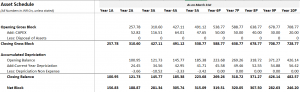Of course, you take the accumulated depreciation number from the asset schedule and complete the netblock calculation in the balance sheet.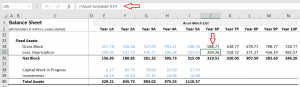With this, we have projected the Fixed assets section in the balance sheet.

In the next chapter, we will discuss the debt schedule.

### Key takeaways from this chapter

• Accumulated depreciation stated in the balance sheet is on a cumulative basis.
• The current year depreciation stated in the P&L is for the year only
• You can use the method of proportions to forecast the depreciation for the year
• You can apply the base rule to forecast the accumulated depreciation
• Netblock = Gross block – accumulated depreciation

1.Tanmay says:

Hello sir, do you know the fact, Zerodha don’t need to invest their money on marketing, because of the trust that Nikhil Sir, Nitin kamath Sir,Karthik Rangappa Sir and all his team created among all the Janta.
( this I can say after listening both brothers on TRS Podcast).

Seriously thanks a lot sir for all this Hardwork that you all are doing consistently. I have started investing a year ago because of this varsity, now i have started Financial Modelling because of this only.
Blessings From My side Sir!!
And one more thing please , provide us some virtual projects or interns ( If possible, No paid chalega XD) so that we can learn more,because at the end what actually matters is ” Kaam Aana Chaiye”

•Karthik Rangappa says:

Thanks for the very kind words, Tanmay. I’m glad you liked the content on Varsity 🙂 About projects, I’ve been trying to nudge ppl to take up tasks in the Financial modelling module. Hopefully, that will help 🙂

2.AZEEM says:

What is the significance of net block?

•Karthik Rangappa says:

Net block = Gross block – Accumulated depreciation.

Basically gives you a sense of how the compnay’s fixed assets are growing.

3.Harsh Dhiman says:

Because of this module principles , i have gained the conviction to stay invested in a company i chose..
Thanks sir

•Karthik Rangappa says:

Happy learning, Harsh!

4.Kamlesh says:

Hello Karthik,
So, I was going through the Asset Schedule of Apollo and there the data is in PP&E instead of Gross Block and also, there is no such Depriciation item in Balance sheet so for that reason what I did is assumed the PP&E in B/s as Net block and used it to reverse it for Gross block using Depriciation given in Income statement.
So this is how it looked:

Opening PP&E :
Less : Depriciation : (Took the Current depriciation amount)
Add – Acquisitions : (Given in Operating cash flow)
Any Changes :
Closing PP&E : (Assumed as Net block)

(But there is a difference in the amount in asset schedule amount and balance sheet amount, so should I write it off balancing the amount and writing it off with “Any changes”)

Sorry for a such a lenghty query but was applying your model method for a competition.
Thankyou again.

•Karthik Rangappa says:

Kamlesh, so if you are considering PP&E as netblock, then why are you deducting depreciation again? Net block is what you get after deducting depreciation right?

5.Shivansh Agarwal says:

the company which I have taken has not given the accumulated depreciation in its balance sheet, the only place where deprecation is mentioned in its financial statements is in p&l statement under expenses

and yea! one more thing the company which I have chosen, in their balance sheet they have not clubbed assets under gross block (there is no such thing like gross block and net block in the balance sheet) they have given each asset and its value separately in the balance sheet so for the gross block what I did was I went deep into the statements and found out the gross value

so what should I do for the accumulated depreciation

•Karthik Rangappa says:

Shivansh, please check the associated notes. They should have mentioned the accumulated depreciation in the balance sheet. Even the gross block.